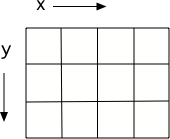# Understanding Image Representation¶

Understanding images requires understanding a set of abstractions:

1. Pictures are made up of little pixels, laid out on an (x,y) grid.

2. Each pixel contains a color.

3. Each color has a red part, a green part, and a blue part.

4. Each color part is actually a number between 0 and 255.

5. If a pixel has red=0, green=0, and blue=0 then the color is black since this is an absence of any color of light.

6. If a pixel as red=255, green=255, and blue=255 then the color is white. Try tilting the bottom of a cd in white light to see the colors in the light.Figure: A grid with horizontal (x) and vertical (y) dimensions

There are some excellent CS Unplugged activities for understanding image and color representation.

Note

Discuss topics in this section with classmates.